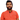# Learn Pain LessHomeOur TeamContact
Python
How to Implement DWave Qbsolve in Python: A Comprehensive GuidePawneshwer Gupta
March 04, 2023
3 min

01
Understanding D-Wave Qbsolve
02
Getting Started with D-Wave Qbsolve in Python
03
Solving the Optimization Problem
04
Step 1: Install the Required Packages
05
Step 2: Import the Required Libraries
06
Step 3: Create a QUBO Matrix
07
Step 4: Solve the QUBO Matrix
08
Step 5: Analyze the Results
09
ConclusionIn this guide, we will provide a comprehensive overview of how to implement DWave Qbsolve in Python. We will cover everything from the basics to more advanced topics, so whether you’re a beginner or an experienced developer, this guide will help you get started with D-Wave Qbsolve.

At present, quantum computing is one of the most rapidly developing fields in the world of computer science. With its unmatched power and speed, it is becoming increasingly popular among businesses, researchers, and developers worldwide. One such tool that has caught the attention of the quantum community is D-Wave Qbsolve, which provides an efficient way of solving complex optimization problems.

D-Wave Qbsolve is a software tool designed to solve optimization problems using quantum computing. It is based on the QUBO (Quadratic Unconstrained Binary Optimization) model, which is a mathematical model that represents optimization problems as a set of binary variables.

One of the key advantages of D-Wave Qbsolve is its ability to solve complex optimization problems more efficiently than classical computers. This is achieved by utilizing the power of quantum annealing, a process that allows the system to explore a vast search space of possible solutions simultaneously.

## Getting Started with D-Wave Qbsolve in Python

Before we start working with D-Wave Qbsolve in Python, we need to install the necessary packages. The following command can be used to install the required packages:

`.css-1baulvz{display:inline-block;}!pip install dwave-ocean-sdk`

Once we have installed the required packages, we can start by importing the necessary modules in Python. The following code demonstrates how to import the required modules:

`from dwave.system.samplers import DWaveSamplerfrom dwave.system.composites import EmbeddingCompositefrom dimod import BinaryQuadraticModel`

With the required modules imported, we can now create a binary quadratic model (BQM), which is the mathematical representation of the optimization problem we want to solve. The following code demonstrates how to create a BQM:

```# Define the variablesvariables = ['x1', 'x2', 'x3']
# Define the coefficients of the quadratic termsquadratic = {('x1', 'x2'): 1.0, ('x2', 'x3'): -2.0, ('x1', 'x3'): 2.0}
# Define the coefficients of the linear termslinear = {'x1': 0.5, 'x2': -1.5, 'x3': 1.0}
# Define the constant termoffset = 0.0

In the above code, we have defined three variables (‘x1’, ‘x2’, and ‘x3’) and their associated coefficients for the quadratic and linear terms. We have also defined the constant term (offset) and created a BQM using the BinaryQuadraticModel class.

## Solving the Optimization Problem

Now that we have created a BQM, we can solve the optimization problem using D-Wave Qbsolve. The following code demonstrates how to solve the BQM using D-Wave Qbsolve:

```# Define the samplersampler = EmbeddingComposite(DWaveSampler())
# Solve the BQMsampleset = sampler.sample(bqm, num_reads=1000)```

In the above code, we have defined the sampler to be the D-Wave system and passed the BQM to the sampler using the sample() method.

#### Setting Up DWave Qbsolve in Python

Now that we have a better understanding of what DWave Qbsolve is and how it works, let’s take a look at how to set it up in Python.

## Step 1: Install the Required Packages

Before we can start using DWave Qbsolve in Python, we need to install the required packages. You can install the packages using pip, the package installer for Python.

`pip install dwave-qbsolv dwave-ocean-sdk`

## Step 2: Import the Required Libraries

After installing the required packages, we need to import the required libraries in our Python script. We will be using `dwave-qbsolv`, `dwavebinarycsp`, and `dimod` libraries in our script.

`from dwave_qbsolv import QBSolvfrom dwavebinarycsp import *import dimod`

## Step 3: Create a QUBO Matrix

Next, we need to create a QUBO matrix. We can do this by defining the variables and the equations that we want to use in our optimization problem. For example, let’s say we want to find the minimum value of the following function:

`f(x,y) = x^2 + 2xy + 3y^2 + 4x + 5y + 6`

We can define the variables and equations as follows:

```csp = dwavebinarycsp.ConstraintSatisfactionProblem(dwavebinarycsp.BINARY)x = csp.add_variable('x')y = csp.add_variable('y')csp.add_constraint(x + y <= 1)csp.add_constraint(x*y == 0)csp.add_constraint(2*y - x <= 1)
qubo = dwavebinarycsp.stitch(csp)```

The resulting `qubo` matrix will be a sparse matrix with the coefficients of the variables in the function.

## Step 4: Solve the QUBO Matrix

After creating the QUBO matrix, we can solve it using DWave Qbsolve. We can do this by passing the `qubo` matrix to the `QBSolv` function and specifying the number of reads that we want to perform.

`response = QBSolv().sample_qubo(qubo, num_reads=100)`

The `response` object will contain the solution to our optimization problem.

## Step 5: Analyze the Results

Finally, we can analyze the results of the optimization problem by looking at the samples returned by the `response` object. Each sample will be a dictionary containing the values of the variables that correspond to the minimum value of the function.

`for sample, energy in response.data(['sample', 'energy']):    print(sample, energy)`

## Conclusion

DWave Qbsolve is a powerful tool that can be used to solve optimization problems. By implementing it in Python, we can easily create and solve optimization problems using the QUBO formulation. We hope that this article has provided you with a better understanding of how to use DWave Qbsolve in Python.

We'll send you the best of our blog just once a month. We promise.

## Tags

D-WaveQbsolveGuide

## Software Developer

Pawneshwer Gupta works as a software engineer who is enthusiastic in creating efficient and innovative software solutions.

Python
Flutter
Laravel
NodeJS

## Related PostsHow to Read a File in Python: A Comprehensive Guide
March 20, 2023
2 min